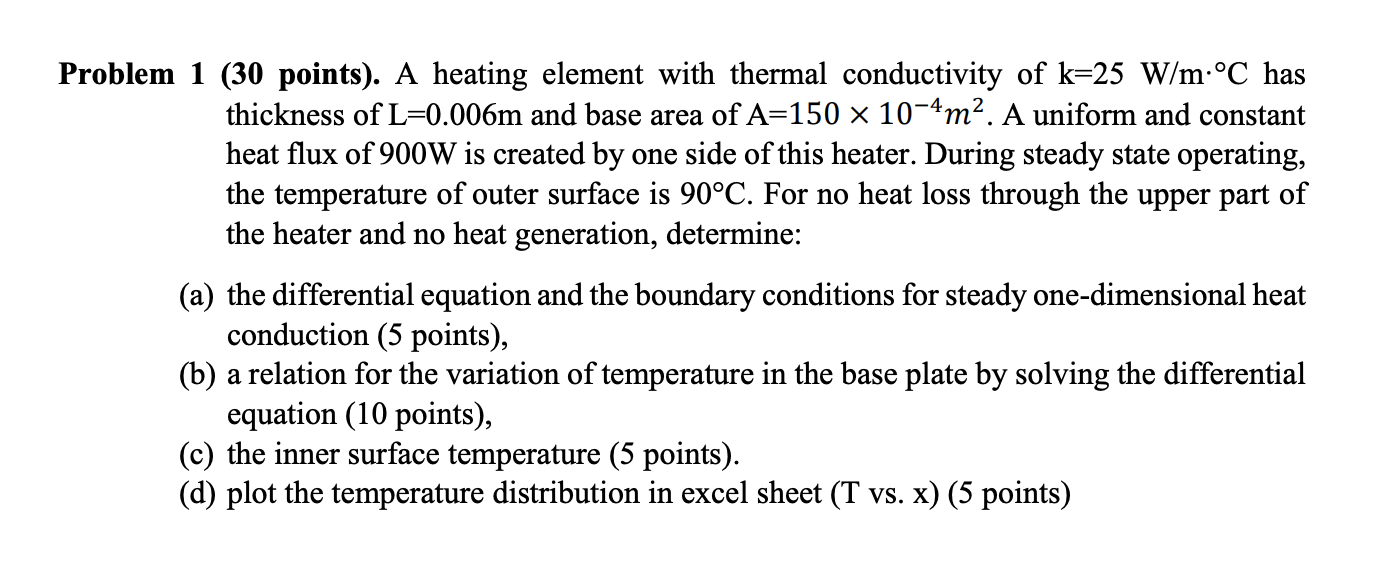Home / Answered Questions / Other / problem-1-30-points-a-heating-element-with-thermal-conductivity-of-k-25-w-m-c-has-thickness-of-l-0-0-aw142

# (Solved): Problem 1 (30 Points). A Heating Element With Thermal Conductivity Of K=25 W/m:Â°C Has Thickness Of ...Problem 1 (30 points). A heating element with thermal conductivity of k=25 W/m:Â°C has thickness of L=0.006m and base area of A=150 x 10-4mÂ². A uniform and constant heat flux of 900W is created by one side of this heater. During steady state operating, the temperature of outer surface is 90Â°Ð¡. For no heat loss through the upper part of the heater and no heat generation, determine: (a) the differential equation and the boundary conditions for steady one-dimensional heat conduction (5 points), (b) a relation for the variation of temperature in the base plate by solving the differential equation (10 points), (c) the inner surface temperature (5 points). (d) plot the temperature distribution in excel sheet (T vs. x) (5 points)

We have an Answer from Expert